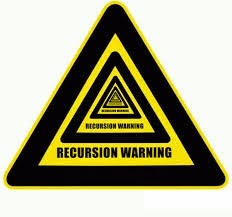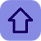# Fibonacci series by Recursion in c

Posted by Tushar Bedekar

### Introduction:-

Recursion is the process of repeating items in a self-similar way. In other words recursion is a process in which a same set of a statement/expressions are executed large no of times in such a manner that after the execution of a certain set of a statement/expression, again the same set of a statement/expressions are being executed until the condition is satisfied. Generally this kind of a process takes place in the in functions in which a function calls itself. The following is the syntax of the recursive function:-
```void recursion()
{
recursion(); /* function calls itself */
}

int main()
{
recursion();
}```Actually most of the programming languages support recursion an C programming language is one of them i.e., a function to call itself. But while using recursion, programmers need to be careful to define an exit condition from the function, otherwise it will go in infinite loop.
Recursive function are very useful to solve many mathematical problems like to calculate factorial of a number, generating Fibonacci series, etc.

### Program me:-

```
/* Fibonacci by Recursion */

#include<stdio.h>int fib(int);

int main()
{
printf("Type any value : ");
printf("\nNth value: %d",fib(getche()-'0'));
return 0;
}

int fib(int n)
{
if(n<=1)
return n;
return(fib(n-1)+fib(n-2));
}```